Olympiad Test Level 1: Quantitative Aptitude & Reasoning- 1

# Olympiad Test Level 1: Quantitative Aptitude & Reasoning- 1

Test Description

## 10 Questions MCQ Test GK Olympiad for Class 7 | Olympiad Test Level 1: Quantitative Aptitude & Reasoning- 1

Olympiad Test Level 1: Quantitative Aptitude & Reasoning- 1 for Class 7 2023 is part of GK Olympiad for Class 7 preparation. The Olympiad Test Level 1: Quantitative Aptitude & Reasoning- 1 questions and answers have been prepared according to the Class 7 exam syllabus.The Olympiad Test Level 1: Quantitative Aptitude & Reasoning- 1 MCQs are made for Class 7 2023 Exam. Find important definitions, questions, notes, meanings, examples, exercises, MCQs and online tests for Olympiad Test Level 1: Quantitative Aptitude & Reasoning- 1 below.
 1 Crore+ students have signed up on EduRev. Have you?
Olympiad Test Level 1: Quantitative Aptitude & Reasoning- 1 - Question 1

### What is the value of 0 - 1 + 2 - 3 + 4 - 5 + 6 - 7 ........... - 17 + 18 - 19 + 20?

Detailed Solution for Olympiad Test Level 1: Quantitative Aptitude & Reasoning- 1 - Question 1

0 - 1 + 2 - 3 + 4 - 5 + 6 - 7...... - 17 + 18 - 19 + 20
= 0 + (-1 + 2) + (-3 + 4) + (-5 + 6) + ...... + (-17 + 18) + (-19 + 20)
= 0 + 1 + 1 + ...... + 1 + 1 = 10

Olympiad Test Level 1: Quantitative Aptitude & Reasoning- 1 - Question 2

### In a box, (3/4)th of the balls are red, (1/6)th are white and the rest are blue. If there are 3 blue balls in the box, how many are red?

Detailed Solution for Olympiad Test Level 1: Quantitative Aptitude & Reasoning- 1 - Question 2

Fraction for blue balls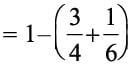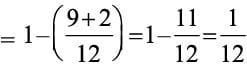Blue balls = 3 {given}.
Now, 1/12 of total balls = 3
⇒ Total number of balls = 3 x 12 = 36
Hence, number of red balls = (3/4)th of 36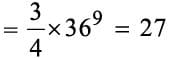Olympiad Test Level 1: Quantitative Aptitude & Reasoning- 1 - Question 3

### An insect crawls up 5 cm every second on a 60 cm vertical rod and then falls down 2 cm over the next second. How many seconds will it take to climb the rod?

Detailed Solution for Olympiad Test Level 1: Quantitative Aptitude & Reasoning- 1 - Question 3

Distance covered in 1st second = 5 cm
Distance covered in 2nd second = - 2 cm
∴ Total distance covered in two seconds
= 5 - 2 = 3 cm
Time taken in climb distance of 3 cm = 2 secs
Distance climbed by insect in 38 secs.
= 38/2 x 3 = 57cm
Distance covered in 39th secs
= 57 + 5
= 62 cm
Which is more than the rod height.
Hence, insect will climb the rod in 39 sec.

Olympiad Test Level 1: Quantitative Aptitude & Reasoning- 1 - Question 4

Calculate the value of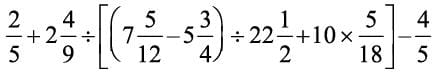Detailed Solution for Olympiad Test Level 1: Quantitative Aptitude & Reasoning- 1 - Question 4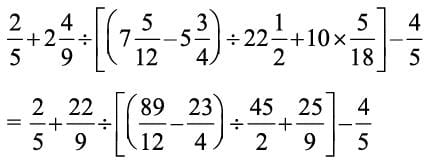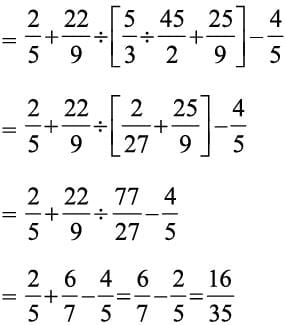Olympiad Test Level 1: Quantitative Aptitude & Reasoning- 1 - Question 5

Directions: The total sales of a company (in crores) for different years are given in the following bar graph. Study the bar graph carefully and answer the following question.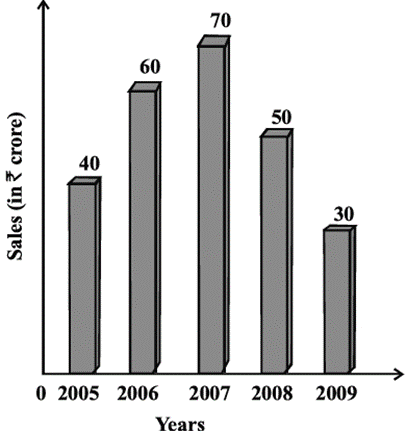Q. In which year the sales of the company was maximum?

Olympiad Test Level 1: Quantitative Aptitude & Reasoning- 1 - Question 6

Directions: The total sales of a company (in crores) for different years are given in the following bar graph. Study the bar graph carefully and answer the following question.Q. In which year the sales of the company was minimum?

Olympiad Test Level 1: Quantitative Aptitude & Reasoning- 1 - Question 7

Directions: The total sales of a company (in crores) for different years are given in the following bar graph. Study the bar graph carefully and answer the following question.Q. The percentage decrease in total sales in year 2009 to that in year 2008 is:

Detailed Solution for Olympiad Test Level 1: Quantitative Aptitude & Reasoning- 1 - Question 7

Sales in year 2008 = 50
Sales in year 2009 = 30
Difference = 50 - 30 = 20
∴ Percentage decrease = 20/50 x 100 = 40%

Olympiad Test Level 1: Quantitative Aptitude & Reasoning- 1 - Question 8

Directions: The total sales of a company (in crores) for different years are given in the following bar graph. Study the bar graph carefully and answer the following question.Q. The average sales during these five years is:

Detailed Solution for Olympiad Test Level 1: Quantitative Aptitude & Reasoning- 1 - Question 8

Average sales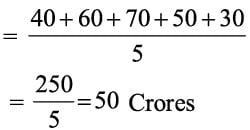Olympiad Test Level 1: Quantitative Aptitude & Reasoning- 1 - Question 9

Directions: The total sales of a company (in crores) for different years are given in the following bar graph. Study the bar graph carefully and answer the following question.Q. The ratio of the total sales in the year 2005 to that in the year 2009 is:

Olympiad Test Level 1: Quantitative Aptitude & Reasoning- 1 - Question 10

Find the mean of the following distribution.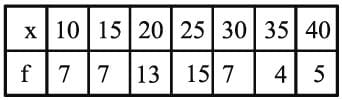Detailed Solution for Olympiad Test Level 1: Quantitative Aptitude & Reasoning- 1 - Question 10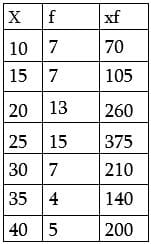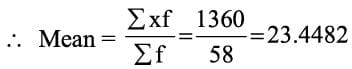## GK Olympiad for Class 7

63 tests
 Use Code STAYHOME200 and get INR 200 additional OFF Use Coupon Code
Information about Olympiad Test Level 1: Quantitative Aptitude & Reasoning- 1 Page
In this test you can find the Exam questions for Olympiad Test Level 1: Quantitative Aptitude & Reasoning- 1 solved & explained in the simplest way possible. Besides giving Questions and answers for Olympiad Test Level 1: Quantitative Aptitude & Reasoning- 1, EduRev gives you an ample number of Online tests for practice

63 tests